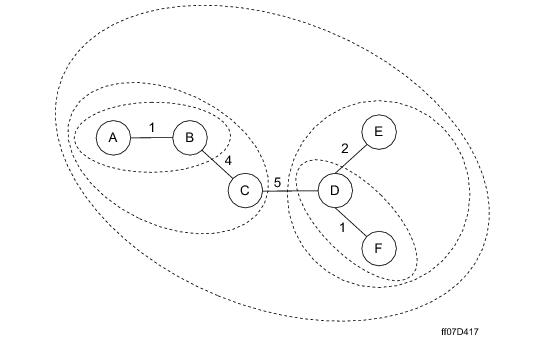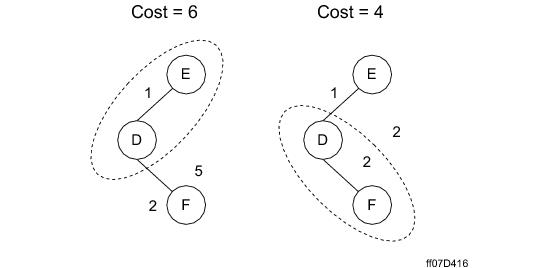## Teradata Database SQL Request and Transaction Processing

prodname
vrm_release
15.10
category
Programming Reference
User Guide
featnum
B035-1142-151K

When it is planning an optimal join sequence for a query, the Optimizer uses a "what if?" method to evaluate the possible sequences for their relative usefulness to the query plan. This "what if?" method is commonly referred to as lookahead in join optimization because the evaluation looks several joins forward to examine the ultimate cost of joining relations in various orders.

Depending on certain well-defined circumstances (see “Five-Join Lookahead Processing of n-Way Joins” on page 383), Teradata Database uses both one‑join and five‑join lookahead methods to determine optimal join plans.

The goal of any n-way join analysis is to reduce the number of join possibilities to a workable subset. The Optimizer search of join space is driven by join conditions. To this end, it uses a recursive greedy algorithm to search and evaluate connected relations. In this context, the term greedy means the search generates the next logical expression for evaluation based on the result of the costing determined by the previous step. From the perspective of search trees, a greedy algorithm always begins at the bottom of the tree and works its way toward the top.

The Optimizer pursues the following strategy in evaluating join sequences for n‑way joins using a one-join lookahead algorithm.

1 Evaluate 3-way joins for the least costly join.

All joins are binary. The term 3-way join is used here to refer to the evaluation of the possible join orders among three relations at a time, not to making a ternary join of the three

2 Commit the first join of the least costly 3-way join.

3 Replace the two source relations used in the join with their resulting joined relation.

4 Loop through the process until only one relation remains.

5 Use a single product join when possible.

The following graphic illustrates the result of an Optimizer analysis of the join space for a 6‑way join. The relative cost of each join is given by an integer within the selected join sequence.These relationships are explicitly stated in the following table.

 This binary join … Has this relative cost … And produces this relation as a result … A - B 1 AB AB - C 4 ABC D - F 1 DF DF - E 2 DFE ABC - DFE 5 ABCDEF

The following graphic illustrates one-join lookahead processing of an n‑way join and how the Optimizer might select one join sequence over another based on their relative costs:The cost relationships among the relations are explicitly stated in the following table.

 This binary join … Has this relative cost … And produces this relation as a result … D - E 1 DE D - F 2 DF DE - F 5 DEF DF - E 2 DEF ABC - DFE 5 ABCDEF

The Optimizer sums the individual binary join costs to produce an overall join cost which it then evaluates to determine the least costly join sequence. For this example, the following join sequence costs are determined.

`   ((DE)F) = 1 + 5 = 6`
` `
`   ((DF)E) = 2 + 2 = 4`

As a result, the second sequence, joining relations D and F first following by joining the resulting relation DF with relation E, is selected for the join plan because it is the less costly join.

Under some circumstances, the Optimizer pursues a second join plan derived from a 5-join lookahead analysis of the join space. This analysis occurs only when any of the following conditions are found to be true.

• The cost of the join plan generated by a 1-join lookahead analysis exceeds a heuristically‑defined threshold.
• The cost of one of the relations being joined exceeds a heuristically‑defined threshold.
• One or more outer joins is required to process the query. Geospatial column terms are not permitted for outer join conditions.
• In such a case, the second join plan is generated using a 5-join lookahead. The relative costs of the two join plans are evaluated and the better plan is used.